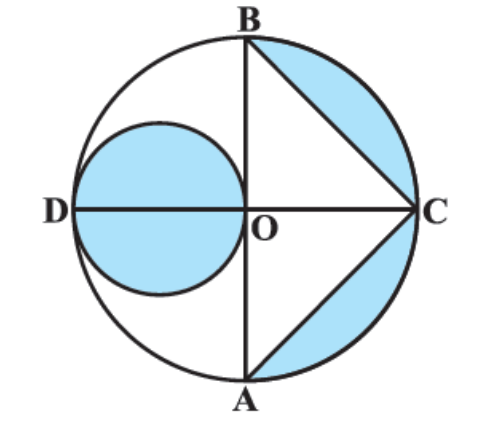In Fig. 12.27, AB and CD are two diameters of a circle (with centre O) perpendicular to each other and OD is the diameter of the smaller circle. If OA = 7 cm, find the area of the shaded region.

# In Fig. 12.27, AB and CD are two diameters of a circle (with centre O) perpendicular to each other and OD is the diameter of the smaller circle. If OA = 7 cm, find the area of the shaded region.Fill Out the Form for Expert Academic Guidance!l

+91

Live ClassesBooksTest SeriesSelf Learning

Verify OTP Code (required)

### Solution:

AB and CD are diameters of the circle with center O

∴ OD = OC = OA = OB = radius of the circle R = 7 cm

∴ AB = 2R = 14 cm

Area of the shaded smaller circular region = πr²

= π (7/2 cm)2

= 22/7 × 7/2 × 7/2 cm2

= 77/2 cm2

= 38.5 cm2

Area of the shaded segment of larger circular region = Area of semicircle ACB - Area of ΔABC

= 1/2 π(OA)2 - 1/2 × AB × OC

= 1/2 πR2 - 1/2 × 2R × R

= 1/2 × 22/7 × 72 - 1/2 × 14 × 7

= 77 - 49

= 28 cm2

Area of the shaded region = Area of the shaded smaller circular region + Area of the shaded segment of larger circular region

= 38.5 cm2 + 28 cm2

= 66.5 cm2

## Related content

 Area of Square Area of Isosceles Triangle Pythagoras Theorem Triangle Formula Perimeter of Triangle Formula Area Formulae Volume of Cone Formula Matrices and Determinants_mathematics Critical Points Solved Examples Type of relations_mathematics+91

Live ClassesBooksTest SeriesSelf Learning

Verify OTP Code (required)